# Welcome to EmpiricalRisks’s documentation!¶

This package provides the basic components for (regularized) empirical risk minization, which is generally formulated as follows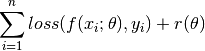As we can see, this formulation involves several components:

• Prediction model: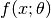, which takes an input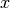and a parameter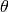and produces an output (say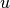).
• Loss function: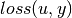, which compares the predicted outputand a desired response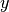, and produces a real value that measuring the loss. Generally, better prediction yields smaller loss.
• Risk model: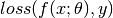, the prediction model and the loss together are referred to as the risk model. When the data x and y are given, the risk model can be considered as a function of theta.
• Regularizer: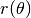is often introduced to regularize the parameter, which, when used properly, can improve the numerical stability of the problem and the generalization performance of the estimated model.

This package provides these components, as well as the gradient computation routines and proximal operators, to support the implementation of various empirical risk minimization algorithms.

All functions in this packages are well optimized and systematically tested.